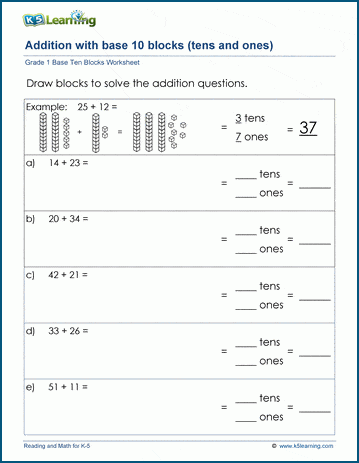# Learning about Tens and Ones Using Base Ten Blocks

Understanding place value starts as early as kindergarten and really ramps up in grade 3, when students are expected to add and subtract within 1,000 using number sense strategies. Understanding place value is not simple, so we start gently in grade 1 by working on tens and ones.

## Recognizing tens and ones

Most kids enter grade 1 with the ability to count to 100 and beyond. The way they think about counting, though, is by ones. 45, 46, 47…

If you ask them how many tens in 45, they may be able to tell you that there are 4 tens. Whether they understand that this number represents 4 tens is a completely different matter.

## Key elements to learning place value

Learning to visualize the groups of tens and ones is an important first step that kids in grade 1 learn. Teachers often work with students on composing and de-composing numbers into their base 10 components to help students grasp this concept.

For example, by ordering sets of blocks into ordered tens and ones: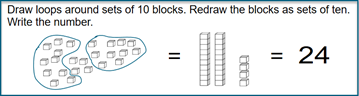Or

Counting using base ten blocks:## Regrouping into blocks of 10 worksheets

We’ve created a series of worksheets to help students work on the first example above.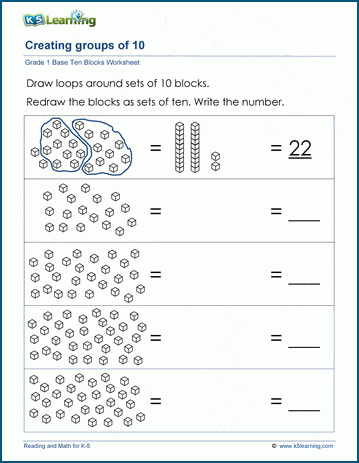## Counting using base 10 blocks

Once they’ve mastered the regrouping concept, they can move on this next set of worksheets: by counting base 10 blocks.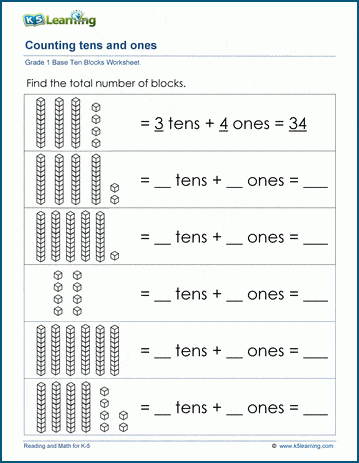## Breaking up a number into tens and ones

In these worksheets, students draw the blocks of tens and ones each number represents.

https://www.k5learning.com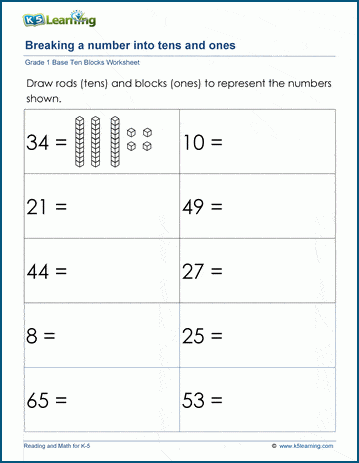## Addition with base 10 blocks worksheets

Once students are familiar with how to construct and deconstruct numbers into tens and ones, they’re ready to work on some simple addition using this learned strategy.

https://www.k5learning.com